• Category
• >Deep Learning

# 5 Common Architectures in Convolution Neural Networks (CNN)

• Rohit Dwivedi
• Jun 07, 2020
• Updated on: Jul 05, 2021## Introduction

In this blog, I will introduce commonly used architectures that are often used for convolution neural networks. If you have gone through the CNN architectures then there are the same fundamentals that are followed by implementing convolutional layers to the input, expanding the number of feature maps and periodically downsampling the spatial dimensions.

While the classical architectures follow assembled convolution layers and modern architectures transverse new and unique ways for building convolutional layers in a way by which there is more structured learning, the same we will understand through the blog.

These different architectures are used by data experts in machine learning models to solve different computer vision problems. They act as rich feature extractors that can be made in use for object detection, image classification, image segmentation and many other tasks. As specifying computer vision context, explore more about computer vision applications.

## Challenges of CNN/ DNN

• The neurons closest to the input layer get slow in learning when the neural networks get too deep that is due to vanishing gradient problems.

• It is seen once in a while that the exploding gradient opposite of the vanishing gradient pannier the learnability of the neuron in the first few layers.

• Initially randomly assigned a weight to the neurons is continued to behold by them.

• The neural network would give poor performance in the production and might give good results in the training. This happens because the role of the first layers of the network is to extract features from the input images.

• The weight should be optimized by the learning process i.e manipulating the weights of the assigned weight to the optimal weights which do not occur due to the problem of vanishing gradient.

• In the case where you have a small volume of data and a number of weights to be learned many then the neural networks get easily overfit.

• While the data is very limited similar to many parameters with low depth when there are many layers with multiple neurons.

• Then this type of model will be poor in production, gets overfitted.

• There the main challenge is to create a neural network overcoming these challenges.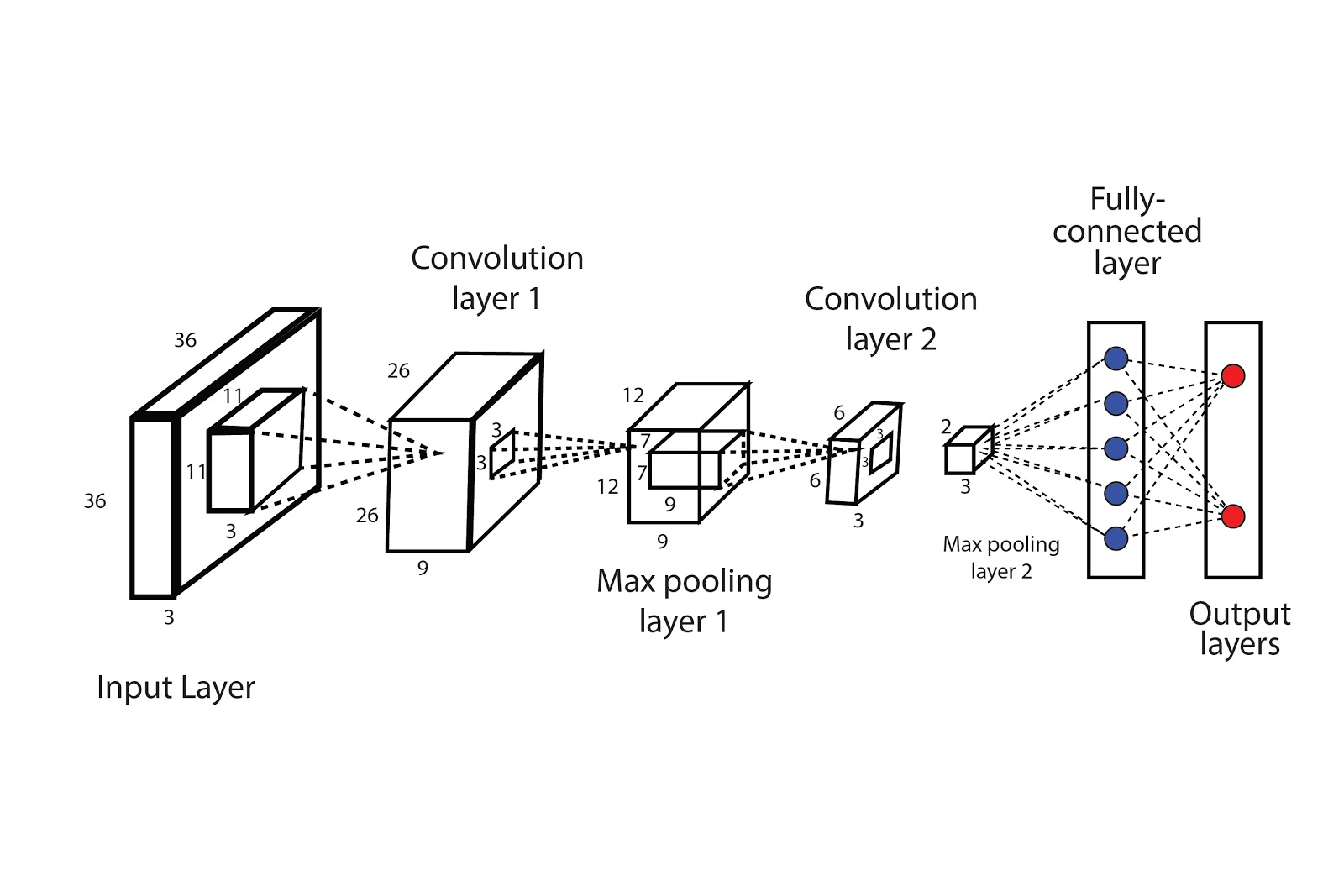Successful CNN Architecture

## 1. LeNet

It is the CNN architecture that is commonly used for hand-written digit recognition (MNIST). It was developed in 1998 by Yann LeCun that had revealed the use of pooling layers and convolution. (Learn more about LeNet-5 from the link provided)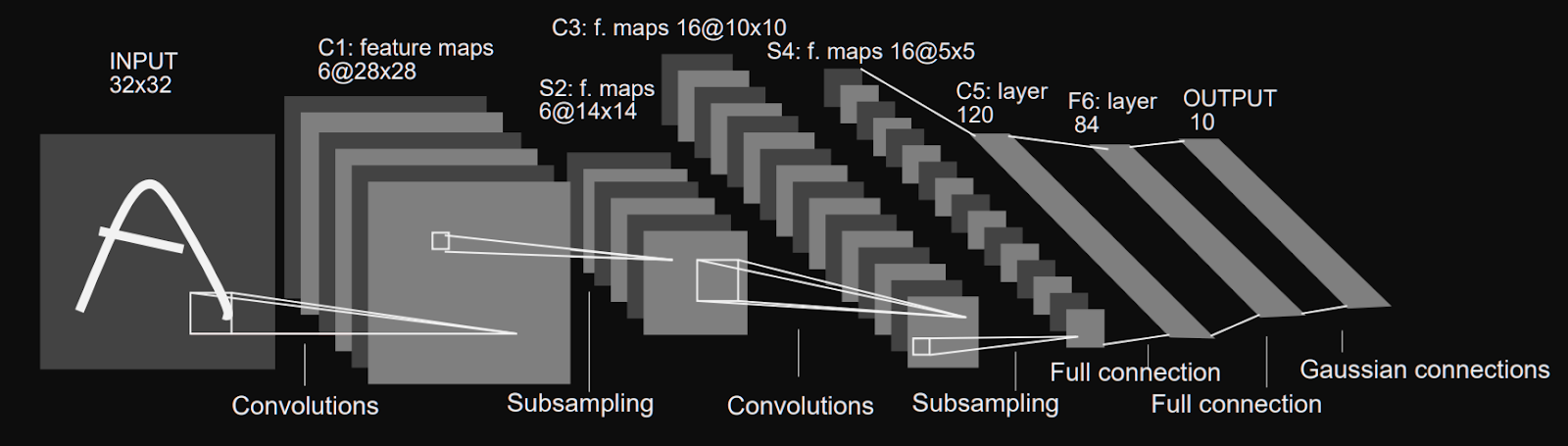LeNet, source

### LeNet Architecture

• LeNet is a 7 layer architecture that excludes the input layer.

• Consisting of 3 convolution layers, a fully connected layer (FC), and an output layer with learnable weights.

• 32×32 pixels image size as the input.

• In the first convolution, a 5×5 kernel that outputs in 6 28×28 feature maps.

• Subsample using  2×2 stride 2 filter that outputs in 6 14×14 feature maps.

• Convolution 16 5×5 kernel that outputs in 16 10×10 feature maps.

• Subsample using  2×2 stride 2 filter that outputs in 16 5×5 feature maps.

• Fully connected dense layers of 120,84,10 layers

### LeNet Features

• First 7 level convolutional network built in 1988 by Lecun.

• Out of 7 layers, 5 layers consisted of two convolutional and three fully connected layers, there 5 layers for learning weights.

• Feature maps keep on diminishing because there is no use of zero paddings anywhere.

• Using tanh as an activation function was not able to make an extensive network due to the vanishing gradient.

• Illustrated the use of subsampling. Max pooling was taken in use.

• 62,000 parameters get trained.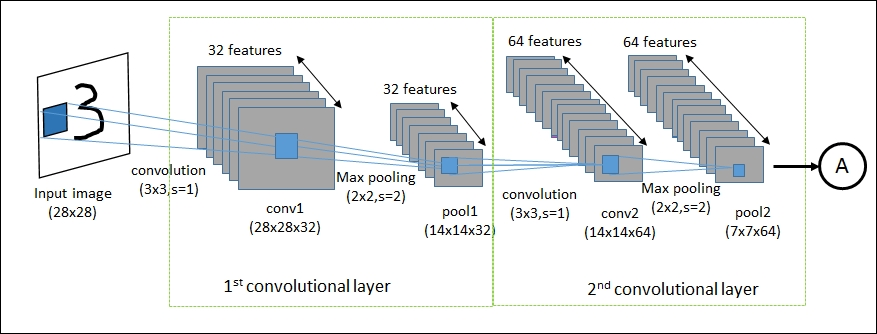LeNet, source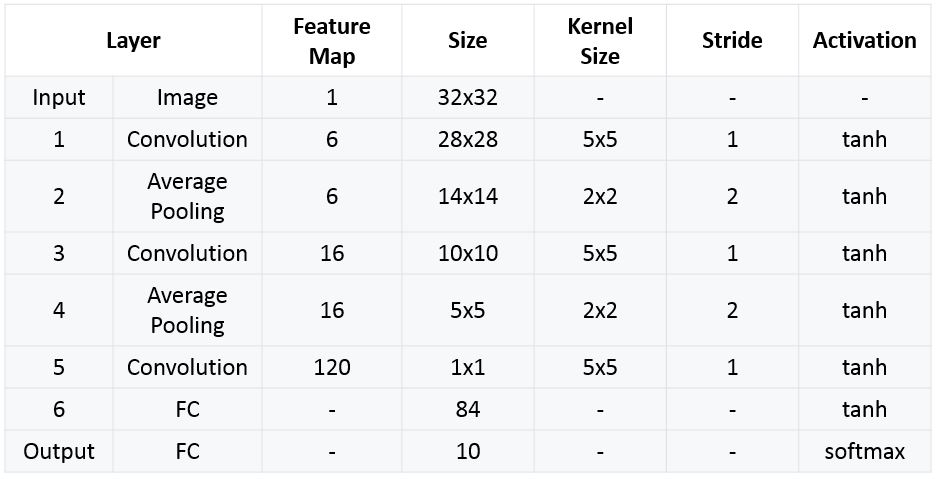LeNet Summary, source

## 2. AlexNet

It was developed in 2012 and was the first architecture that lowered down the top-5% error from 26% to 15.3% in ImageNet Classification.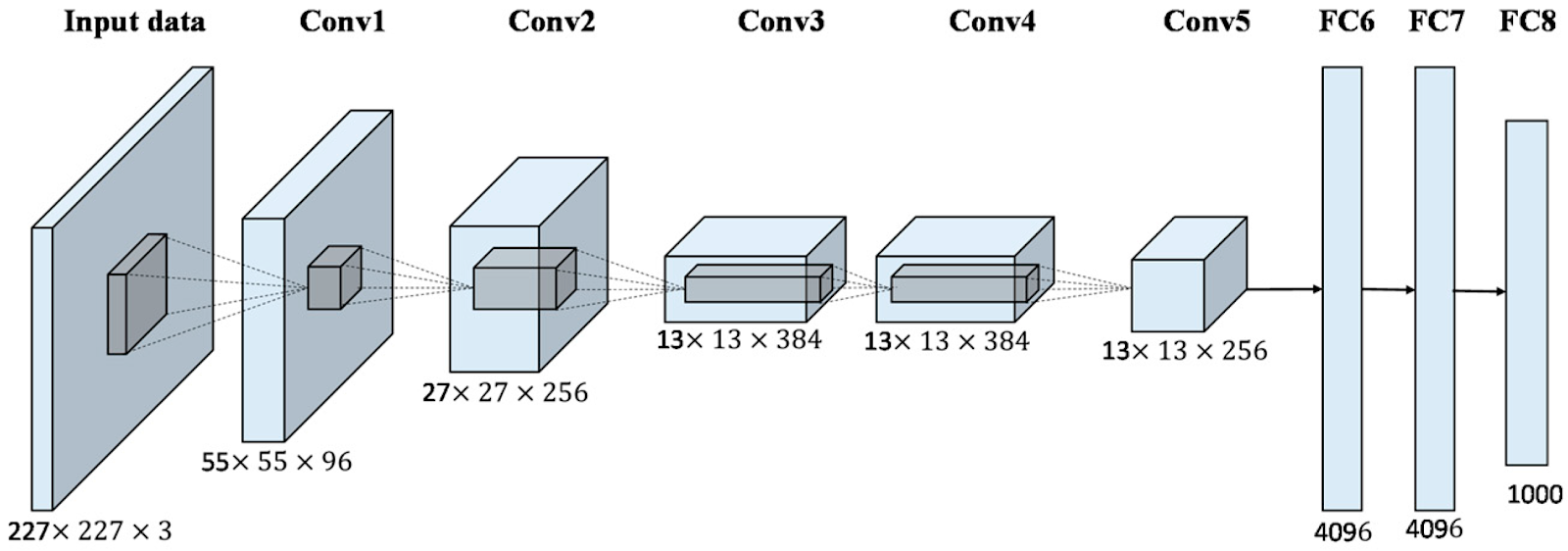AlexNet | Source

### AlexNet Architecture

• Comprises 5 convolutional layers and 3 dense layers.

• There were two news concepts with these 8 layers that were introduced that are MaxPooling and the use of the ReLu Activation function.

### AlexNet Features

• Made use of Rectified Linear Unit (ReLu) as an activation function instead of Tanh or sigmoid for the non-linear part. It trains faster as it overcomes the problem of vanishing gradients.

• Use of the Dropout layer after every dense layer to avoid over-fitting.

• The function of the dropouts is to switch off the activation.

• In the first two convolution layers, large filter was used i.e (11X11 and 5X5)

• With momentum SGS optimizer was used.

• Data augmentation was done to enhance the data for training.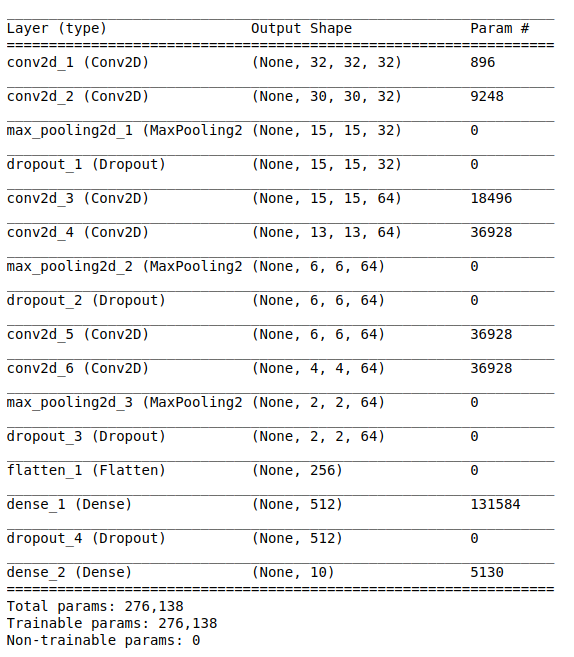AlexNet Summary

## 3. VGG16

A deep convolutional layer network that has 13 convolutional and 3 dense layers, picking up the ReLu tradition from AlexNet. The architecture was developed by the Visual Geometry Group (VGG).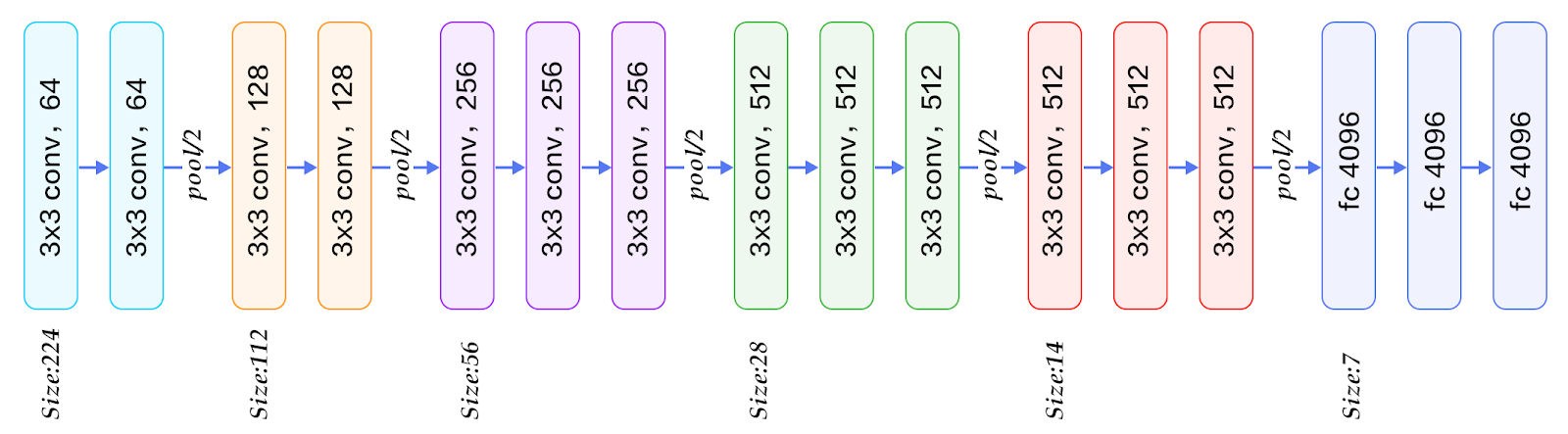VGG16, source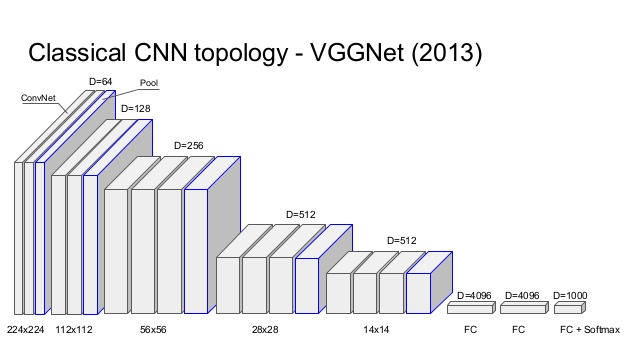VGGNet, source

### VGG Architecture

• VGG had very deep layers and small size filters (2×2 and 3×3)

• Alexnet did not have that deep layers architecture and also used large filter sizes.

• Utilize storage of about 500MB and comprise 138M parameters.

### VGG Features

• Kernel size = 3×3, stride = 1×1, padding = same, the configuration of each convolutional layer. The only changes that were present were the number of filters.

• To retain the feature maps across blocks used Padding permitted an increase in layers.

• Windows size = 2×2 and stride = 2×2, the max pool layer configuration. Therefore the size of the image is halved at every pool layer.

• RGB image as an input image of 224× 224 pixels. Therefore, input size = 224×224×3.

• Training of the model was done in different stages.

• Train shallow network

• Talke weights from shallow network to larger

• Train the new layers.

• The training was done in stages so as to overcome the problem of vanishing/exploding gradients.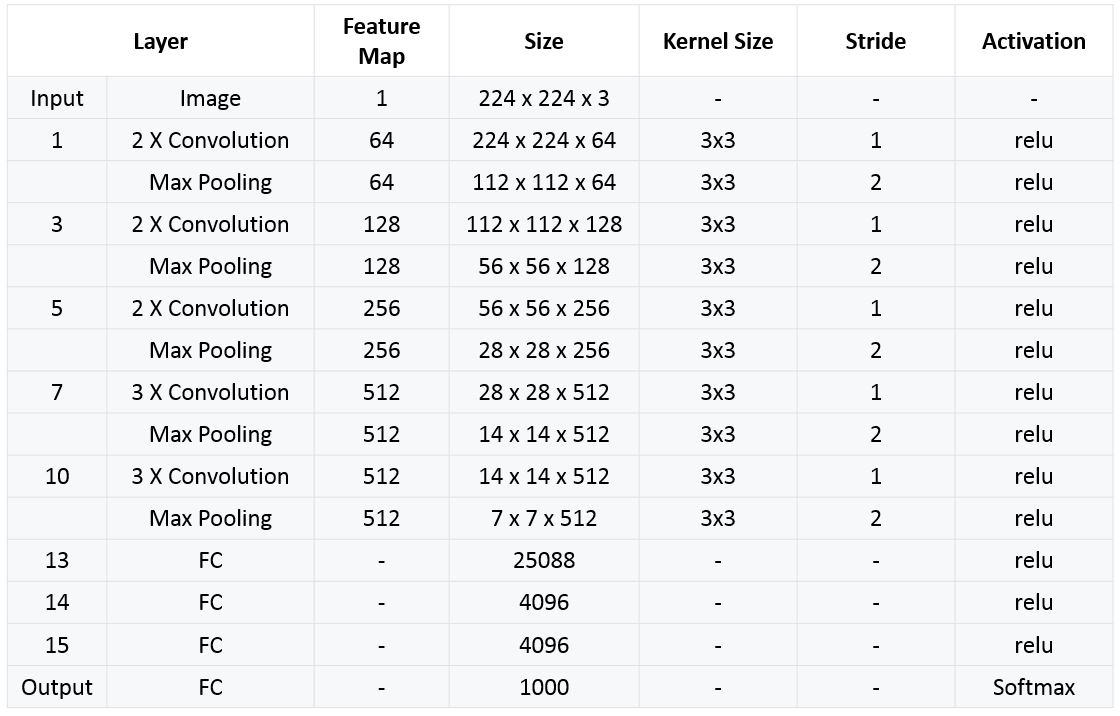VGGNet, source

(Suggest blog: Introduction to Perceptron Model in Machine Learning

Architecture developed by Google was declared as the winner of the ILSVRC 2014 competition also called GoogleNet (Inception V1). It attained a top-5 error percentage of 6.67%.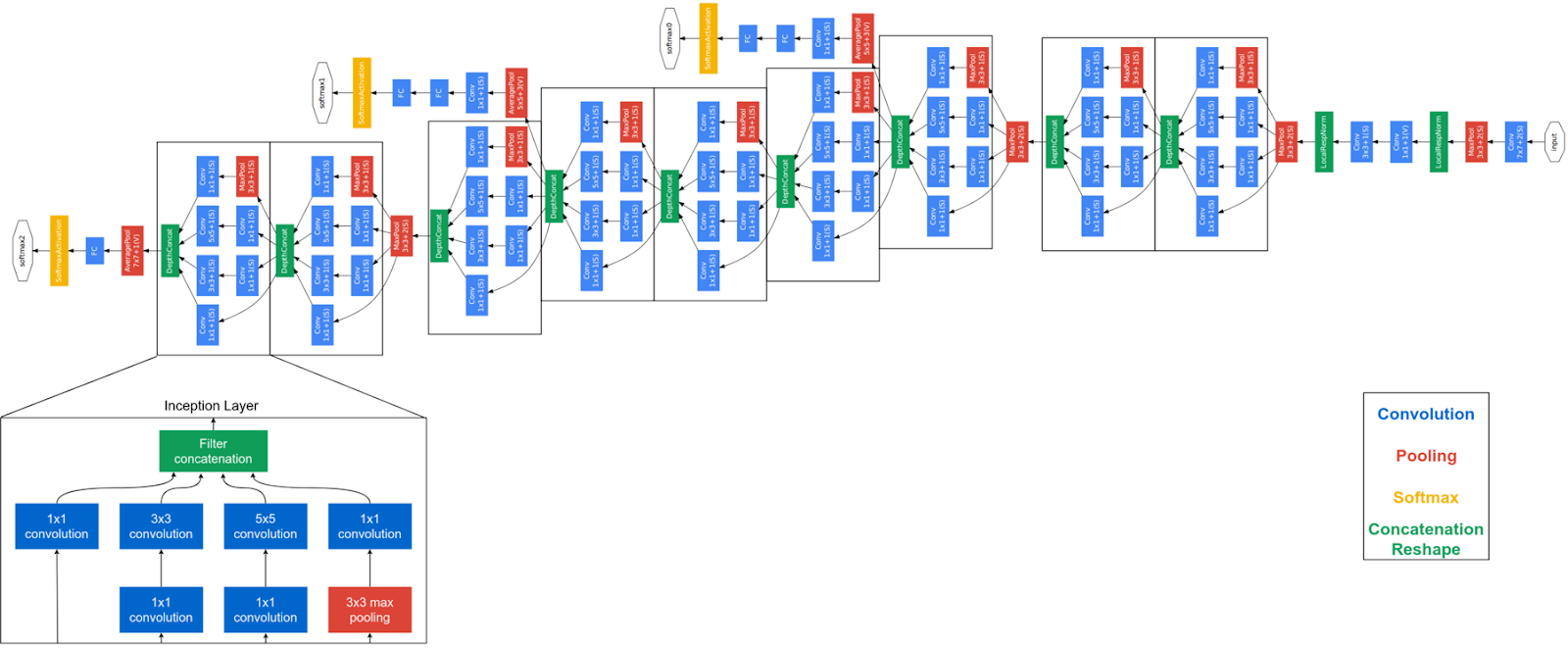Implementation of an element labelled as “inception module” and “auxiliary classifier” along with softmax activation to escalate the gradient signal that gets propagated back and provided additional regularization. As discussing regularization context, explore about L1 and L2 regularization in ML.

• Made use of batch normalization, image distortions.

• The inception module is based on many very small convolutions so as to lower down the number of parameters.

• This architecture has 22 deep CNN layers.

• The number of parameters were lowered down to 4M from 60M (AlexNet).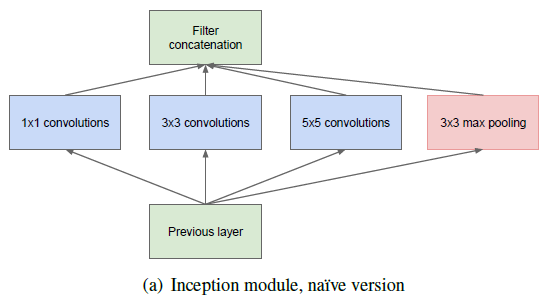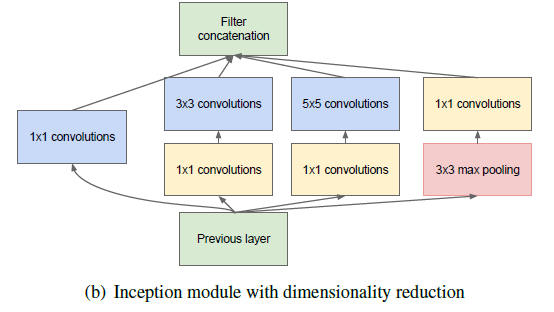Inception module: naive version & inception module dimensionality reduction

• Input signal is emulated and then fed to four different layers.

• ReLu activation function is used by all the convolution layers.

• Different kernel sizes are used by the second set of layers.

• At different scales detaining patterns.

• The stride of 1 and padding = same is used by each single layer.

• Resulting in dimensions of the output alik to dimensions of input.

• This makes it possible to chain all the output with the depth dimension in the final depth concat layer.

## 5. ResNet

It is the deepest convolution network to this day comprising 151 layers that won the 2015 ILSVRC. It lowered down the top 5-error rate of 3.57% that is lower than the human error on top 5. This happened due to the Residual network that was used by Microsoft. They come up with a novel approach called “Skip Connections”.

### Resnet Architecture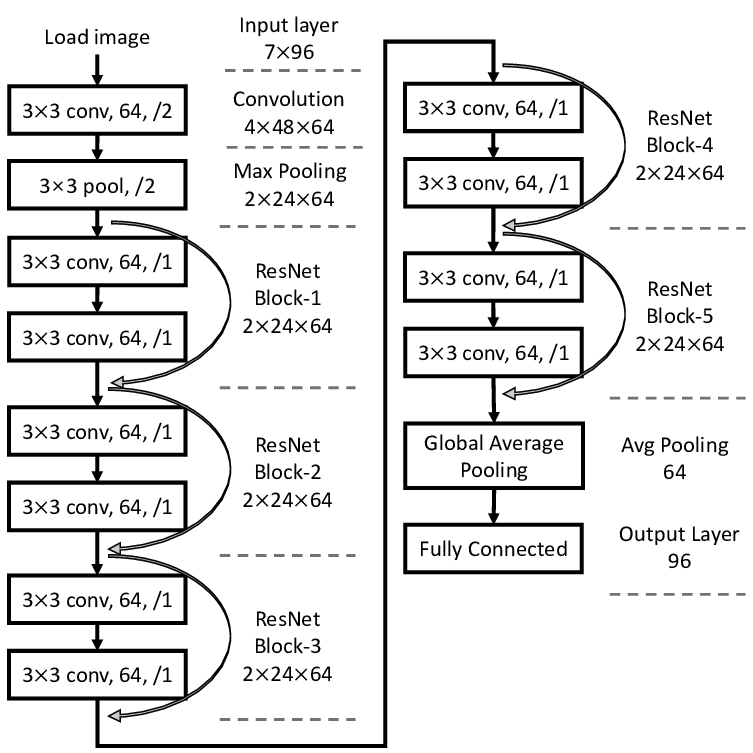ResNet Architecture

### ResNet Features / Vanishing gradient

• In Neural Networks till the first layer, the error gradient gets computed at the end which gets backpropagated through previous layers.

• The chain rule that multiplies the error gradient with multiple terms as it propagates back.

• There can be multiplication with fractions at different points when there is a long chain.

• The outcome gets smaller and smaller when many small fractions get multiplied.

• The first layers don’t get to update their coefficient because they get a very small gradient to work.

• The training gets very slow, moreover, the gradient gets zero meaning no changes in the parameters.

ResNet uses identify functions to backpropagate the gradients. The gradient would not be affected as it is multiplied by 1. This is the concept of ResNet, it mounds these residual blocks together where gradients are preserved using identity function.

## Conclusion

In this blog, I have introduced you to different image classification architectures that were presented in the ImageNet competition. These include LeNet, AlexNet, VGG16, GoogleNet/ Inception and ResNet. These are the benchmark models that are used today for image classifications. There are different techniques of using these models by Transfer learning that would be covered in the next blog.

You can read more about these architectures and many others here. In this Keras API, you will find the documentation that is given for these architectures.